Name:    Quiz 9.1-9.2

Multiple Choice
Identify the choice that best completes the statement or answers the question.

1.

There are 16 sixth graders, 11 seventh graders, and 9 eighth graders in a gym class. The gym teacher randomly selects one student to collect balls. In how many ways can choosing not a seventh grader occur?
 a. 11 ways c. 25 ways b. 20 ways d. 36 ways

2.

The possible rolls on a number cube are 1, 2, 3, 4, 5, and 6. Which word best describes the likelihood of rolling not an 8?
 a. equally likely c. impossible b. certain d. likely

3.

A bucket contains 20 baseballs. Some are regular baseballs and some are soft baseballs. By randomly grabbing a baseball from the bucket, you are equally likely to get a regular baseball or a soft baseball. How many soft baseballs are in the bucket?
 a. 10 c. 13 b. 0 d. 20

Use the spinner to determine the theoretical probability of the event.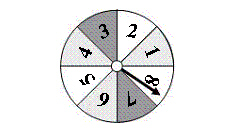4.

Spinning a 3
 a.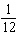c.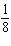b.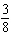d.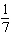5.

Spinning a number less than 5
 a.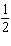c.b.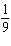d.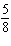6.

Spinning a number less than 11
 a. 1 c.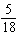b.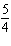d.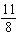7.

Spinning an even number
 a.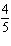c.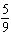b.d.8.

Spinning a multiple of 2
 a.c.b.d.9.

Spinning a multiple of 3
 a.c.b.d.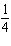10.

Spinning a multiple of 4
 a.c.b.d.11.

You shuffle a deck of 26 cards representing the letters of the alphabet. You randomly select a card from the deck. What is the theoretical probability that the card represents one of the letters in the word “mathematics”?
 a.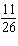c.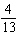b.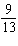d.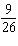Numeric Response

1.

You shuffle a deck of 26 cards representing the letters of the alphabet. You randomly select a card from the deck. What is the theoretical probability that the card represents one of the letters in the word “trampoline”?

You randomly choose one of the tiles shown below. Find the favorable outcomes of the event.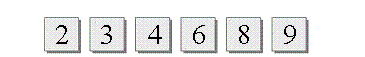1.

Choosing a number divisible by 3

A number cube is rolled. Determine if the game is fair. If it is not fair, who has the greater probability of winning?

2.

You win if the number is greater than 4. Your friend wins if the number is less than 5.